# 8 5 Volume of Prisms and Cylinders Warm

• Slides: 27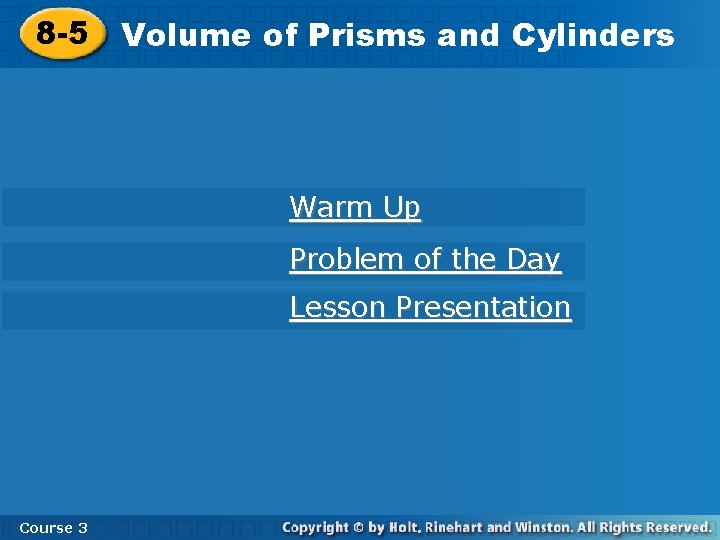8 -5 Volume of Prisms and Cylinders Warm Up Problem of the Day Lesson Presentation Course 38 -5 Volume of Prisms and Cylinders Warm Up Find the area of each figure described. Use 3. 14 for . 1. a triangle with a base of 6 feet and a height of 3 feet 9 ft 2 2. a circle with radius 5 in. 78. 5 ft 2 Course 38 -5 Volume of Prisms and Cylinders Problem of the Day You are painting identical wooden cubes red and blue. Each cube must have 3 red faces and 3 blue faces. How many cubes can you paint that can be distinguished from one another? only 2 Course 3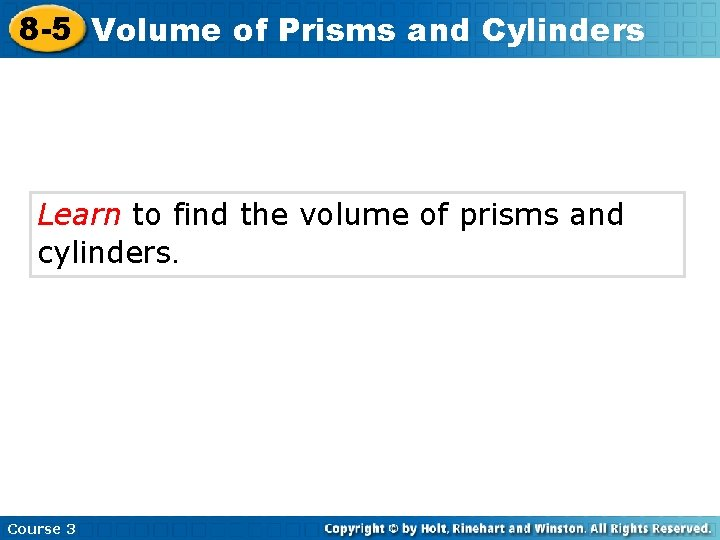8 -5 Volume of Prisms and Cylinders Learn to find the volume of prisms and cylinders. Course 38 -5 Volume Insert Lesson Title Here of Prisms and Cylinders Vocabulary cylinder prism Course 3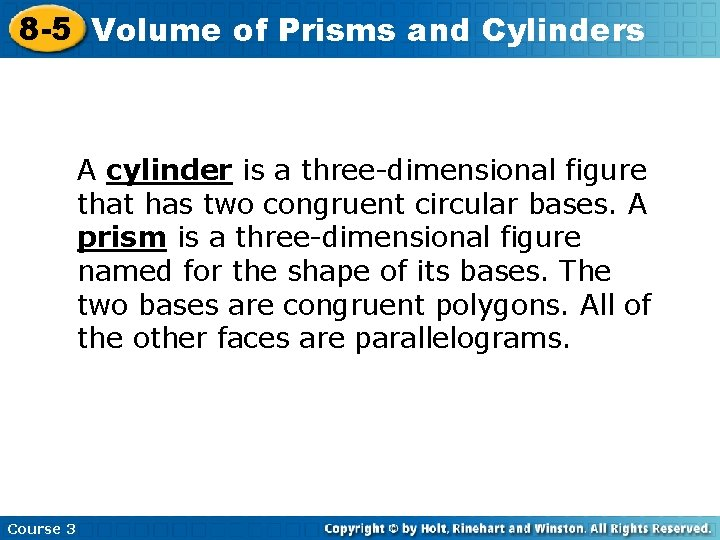8 -5 Volume of Prisms and Cylinders A cylinder is a three-dimensional figure that has two congruent circular bases. A prism is a three-dimensional figure named for the shape of its bases. The two bases are congruent polygons. All of the other faces are parallelograms. Course 38 -5 Volume of Prisms and Cylinders Rectangular prism Triangular prism Height Course 3 Height Base Cylinder Base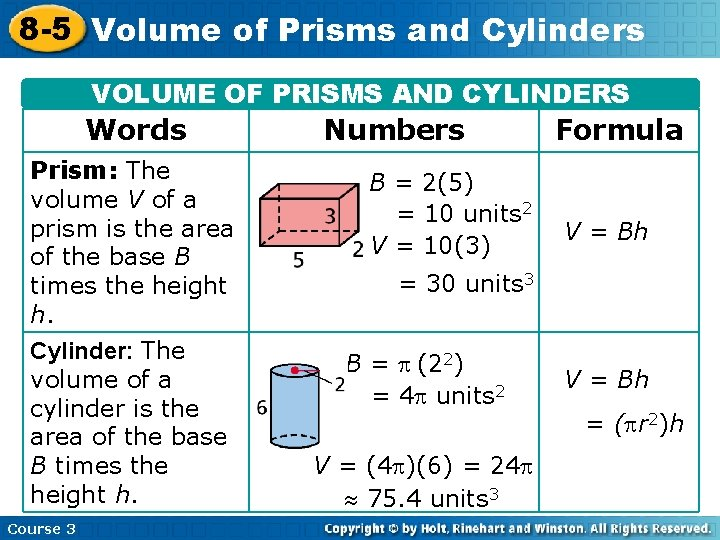8 -5 Volume of Prisms and Cylinders VOLUME OF PRISMS AND CYLINDERS Words Prism: The volume V of a prism is the area of the base B times the height h. Cylinder: The volume of a cylinder is the area of the base B times the height h. Course 3 Numbers B = 2(5) = 10 units 2 V = 10(3) Formula V = Bh = 30 units 3 B = (22) = 4 units 2 V = (4 )(6) = 24 75. 4 units 3 V = Bh = ( r 2)h8 -5 Volume of Prisms and Cylinders Remember! Area is measured in square units. Volume is measured in cubic units. Course 3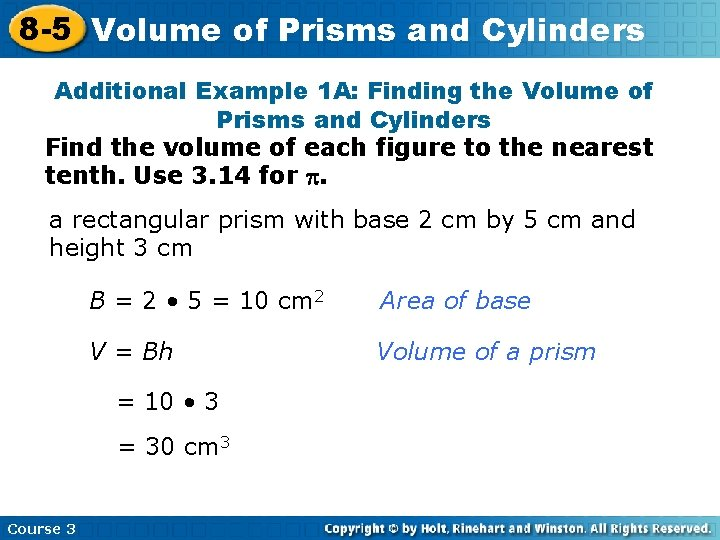8 -5 Volume of Prisms and Cylinders Additional Example 1 A: Finding the Volume of Prisms and Cylinders Find the volume of each figure to the nearest tenth. Use 3. 14 for . a rectangular prism with base 2 cm by 5 cm and height 3 cm B = 2 • 5 = 10 cm 2 Area of base V = Bh Volume of a prism = 10 • 3 = 30 cm 3 Course 38 -5 Volume of Prisms and Cylinders Additional Example 1 B: Finding the Volume of Prisms and Cylinders Find the volume of the figure to the nearest tenth. Use 3. 14 for . 4 in. B = (42) = 16 in 2 Area of base V = Bh 12 in. = 16 • 12 Volume of a cylinder = 192 602. 9 in 3 Course 38 -5 Volume of Prisms and Cylinders Additional Example 1 C: Finding the Volume of Prisms and Cylinders Find the volume of the figure to the nearest tenth. Use 3. 14 for . 1 • 6 • 5 = 15 ft 2 Area of base 2 V = Bh Volume of a prism = 15 • 7 B= 5 ft 7 ft 6 ft Course 3 = 105 ft 3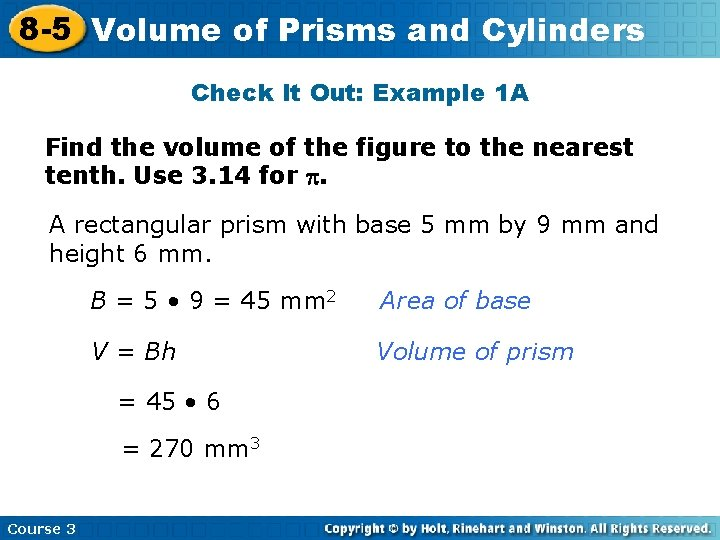8 -5 Volume of Prisms and Cylinders Check It Out: Example 1 A Find the volume of the figure to the nearest tenth. Use 3. 14 for . A rectangular prism with base 5 mm by 9 mm and height 6 mm. B = 5 • 9 = 45 mm 2 Area of base V = Bh Volume of prism = 45 • 6 = 270 mm 3 Course 3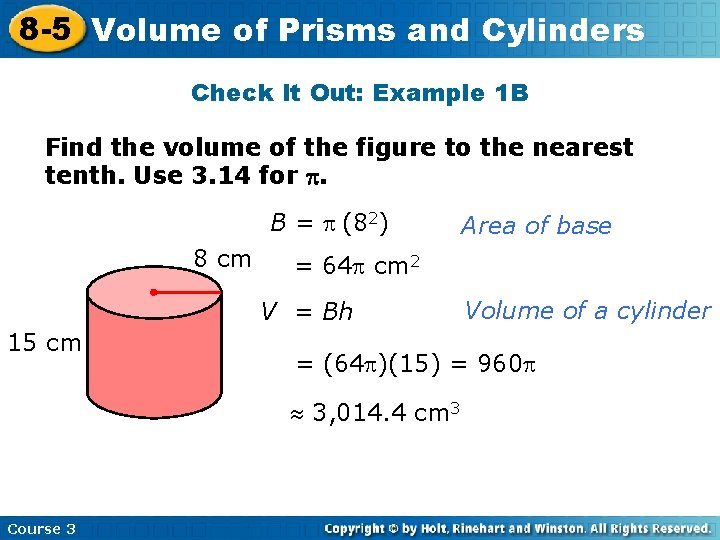8 -5 Volume of Prisms and Cylinders Check It Out: Example 1 B Find the volume of the figure to the nearest tenth. Use 3. 14 for . B = (82) 8 cm = 64 cm 2 V = Bh 15 cm Volume of a cylinder = (64 )(15) = 960 3, 014. 4 cm 3 Course 3 Area of base8 -5 Volume of Prisms and Cylinders Check It Out: Example 1 C Find the volume of the figure to the nearest tenth. Use 3. 14 for . 1 B= • 12 • 10 2 = 60 ft 2 10 ft V = Bh 14 ft 12 ft Course 3 = 60(14) = 840 ft 3 Area of base Volume of a prism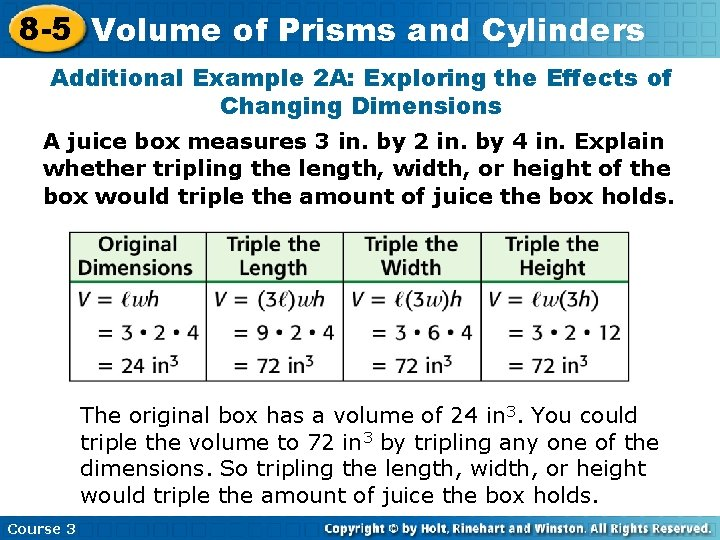8 -5 Volume of Prisms and Cylinders Additional Example 2 A: Exploring the Effects of Changing Dimensions A juice box measures 3 in. by 2 in. by 4 in. Explain whether tripling the length, width, or height of the box would triple the amount of juice the box holds. The original box has a volume of 24 in 3. You could triple the volume to 72 in 3 by tripling any one of the dimensions. So tripling the length, width, or height would triple the amount of juice the box holds. Course 3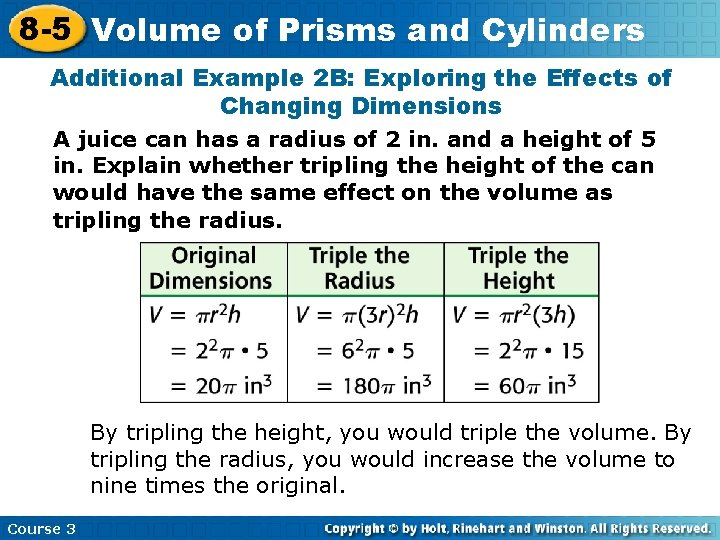8 -5 Volume of Prisms and Cylinders Additional Example 2 B: Exploring the Effects of Changing Dimensions A juice can has a radius of 2 in. and a height of 5 in. Explain whether tripling the height of the can would have the same effect on the volume as tripling the radius. By tripling the height, you would triple the volume. By tripling the radius, you would increase the volume to nine times the original. Course 3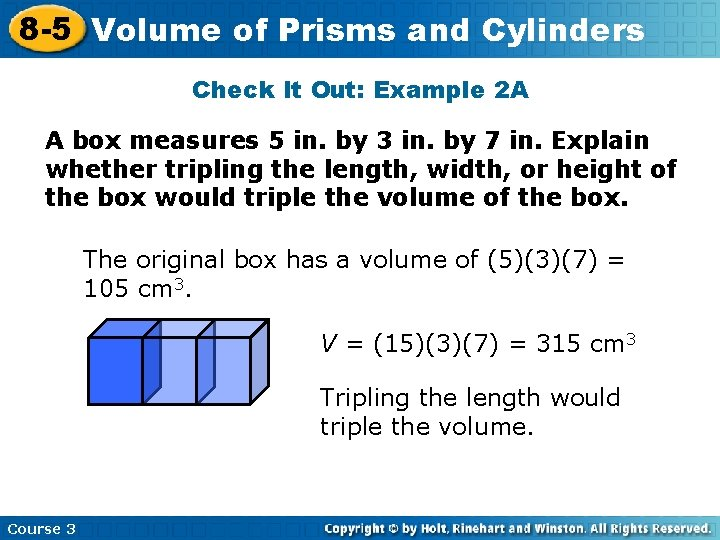8 -5 Volume of Prisms and Cylinders Check It Out: Example 2 A A box measures 5 in. by 3 in. by 7 in. Explain whether tripling the length, width, or height of the box would triple the volume of the box. The original box has a volume of (5)(3)(7) = 105 cm 3. V = (15)(3)(7) = 315 cm 3 Tripling the length would triple the volume. Course 38 -5 Volume of Prisms and Cylinders Check It Out: Example 2 A Continued The original box has a volume of (5)(3)(7) = 105 cm 3. V = (5)(3)(21) = 315 cm 3 Tripling the height would triple the volume. Course 3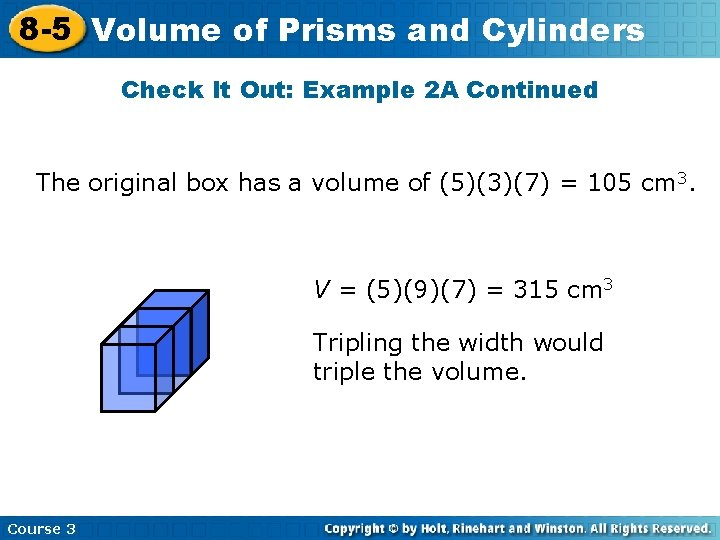8 -5 Volume of Prisms and Cylinders Check It Out: Example 2 A Continued The original box has a volume of (5)(3)(7) = 105 cm 3. V = (5)(9)(7) = 315 cm 3 Tripling the width would triple the volume. Course 3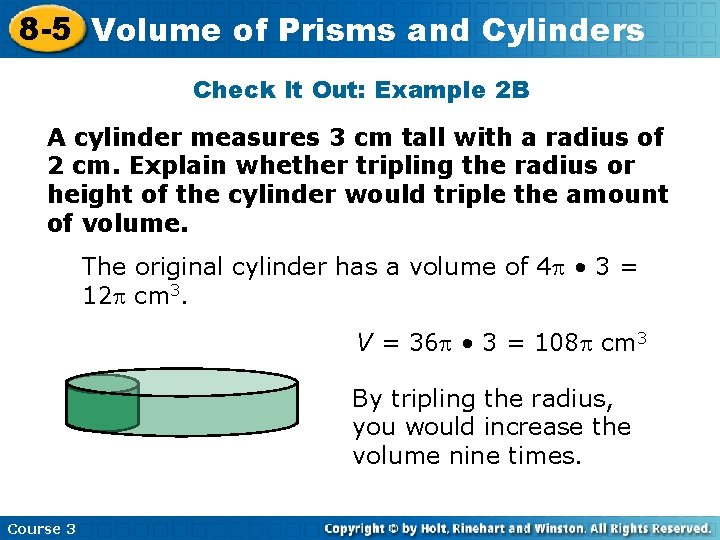8 -5 Volume of Prisms and Cylinders Check It Out: Example 2 B A cylinder measures 3 cm tall with a radius of 2 cm. Explain whether tripling the radius or height of the cylinder would triple the amount of volume. The original cylinder has a volume of 4 • 3 = 12 cm 3. V = 36 • 3 = 108 cm 3 By tripling the radius, you would increase the volume nine times. Course 3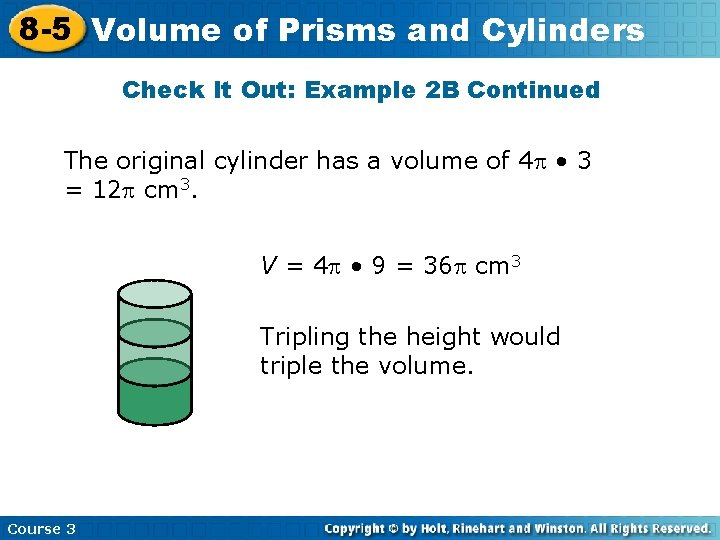8 -5 Volume of Prisms and Cylinders Check It Out: Example 2 B Continued The original cylinder has a volume of 4 • 3 = 12 cm 3. V = 4 • 9 = 36 cm 3 Tripling the height would triple the volume. Course 3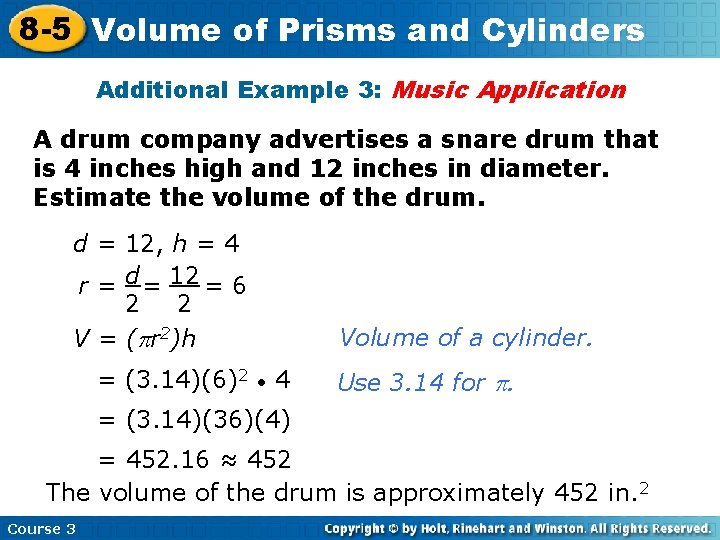8 -5 Volume of Prisms and Cylinders Additional Example 3: Music Application A drum company advertises a snare drum that is 4 inches high and 12 inches in diameter. Estimate the volume of the drum. d = 12, h = 4 r = d = 12 = 6 2 2 V = ( r 2)h = (3. 14)(6)2 Volume of a cylinder. • 4 Use 3. 14 for . = (3. 14)(36)(4) = 452. 16 ≈ 452 The volume of the drum is approximately 452 in. 2 Course 3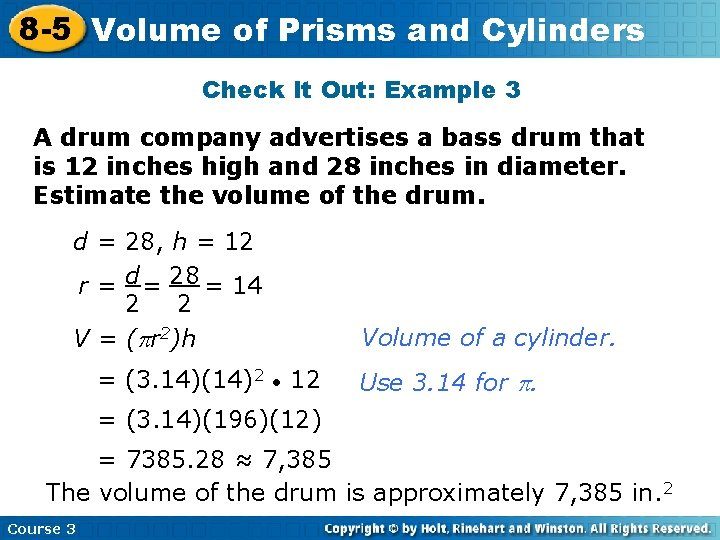8 -5 Volume of Prisms and Cylinders Check It Out: Example 3 A drum company advertises a bass drum that is 12 inches high and 28 inches in diameter. Estimate the volume of the drum. d = 28, h = 12 r = d = 28 = 14 2 2 V = ( r 2)h = (3. 14)(14)2 Volume of a cylinder. • 12 Use 3. 14 for . = (3. 14)(196)(12) = 7385. 28 ≈ 7, 385 The volume of the drum is approximately 7, 385 in. 2 Course 3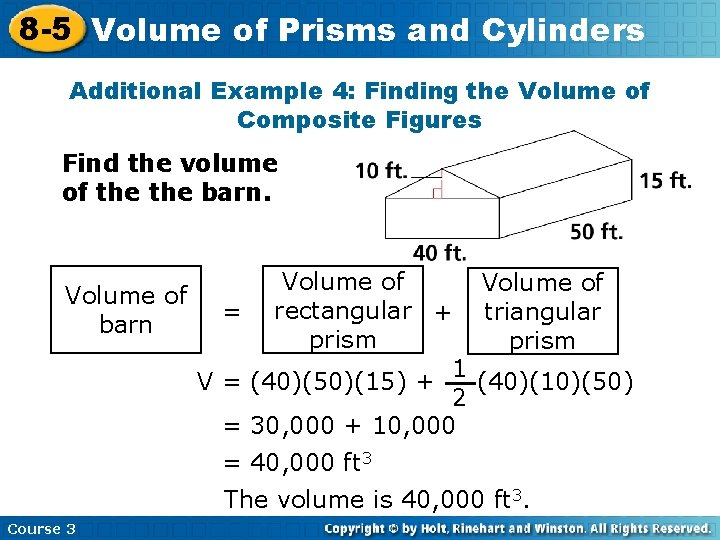8 -5 Volume of Prisms and Cylinders Additional Example 4: Finding the Volume of Composite Figures Find the volume of the barn. Volume of = rectangular + triangular barn prism 1 V = (40)(50)(15) + (40)(10)(50) 2 = 30, 000 + 10, 000 = 40, 000 ft 3 The volume is 40, 000 ft 3. Course 38 -5 Volume of Prisms and Cylinders Check It Out: Example 4 Find the volume of the house. Volume of house Volume of rectangular + prism = = 5 ft = 4 ft 8 ft Course 3 3 ft Volume of triangular prism 1 (8)(3)(4) + (5)(8)(3) 2 96 + V = 156 ft 3 60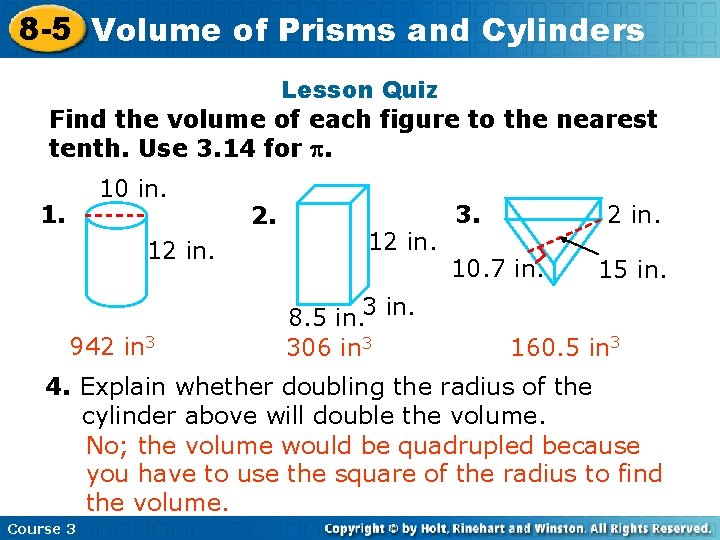8 -5 Volume Insert Lesson of Prisms Title and Here Cylinders Lesson Quiz Find the volume of each figure to the nearest tenth. Use 3. 14 for . 10 in. 12 in. 942 in 3 2. 12 in. 8. 5 in. 306 in 3 3. 2 in. 10. 7 in. 15 in. 160. 5 in 3 4. Explain whether doubling the radius of the cylinder above will double the volume. No; the volume would be quadrupled because you have to use the square of the radius to find the volume. Course 3